Homework Help Question & Answers

# principles of chemistry 122 6. A bottle of wine contains 750.0 mL of wine. If the...

principles of chemistry 122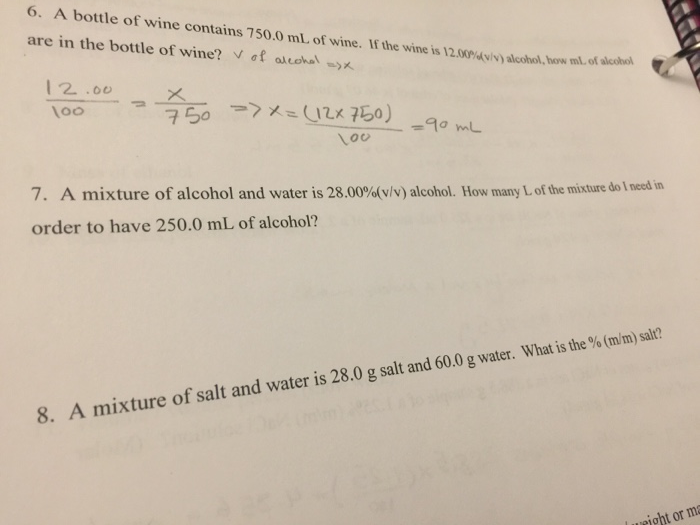6. A bottle of wine contains 750.0 mL of wine. If the wine is 12.00% are in the bottle of wine? V of alcohol ->X ) alcohol, bow ml of alice 12 .00 100 150 >> x=(12*50) 90 mL loo 7. A mixture of alcohol and water is 28.00%(v/v) alcohol. How many of the mixture do I need in order to have 250.0 mL of alcohol? 8. A mixture of salt and water is 28.0 g salt and 60.0 g water. What is the % (m/m) salt? I might or me

#### Homework Answers

Answer #1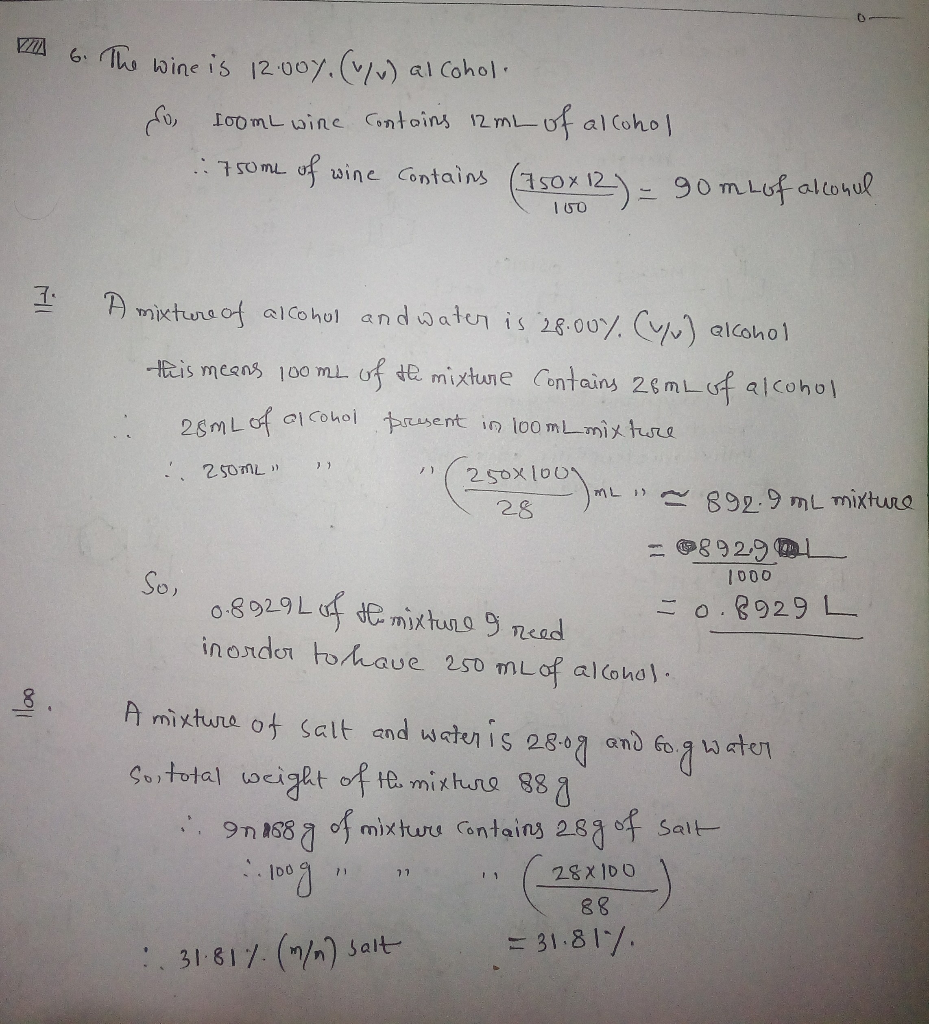Know the answer?
Your Answer:

#### Post as a guest

Your Name:

What's your source?

#### Earn Coin

Coins can be redeemed for fabulous gifts.

Not the answer you're looking for? Ask your own homework help question. Our experts will answer your question WITHIN MINUTES for Free.
Similar Homework Help Questions
• ### A bottle of wine is 14.5% abv (v/v%). If the wine bottle contains 750 mL of...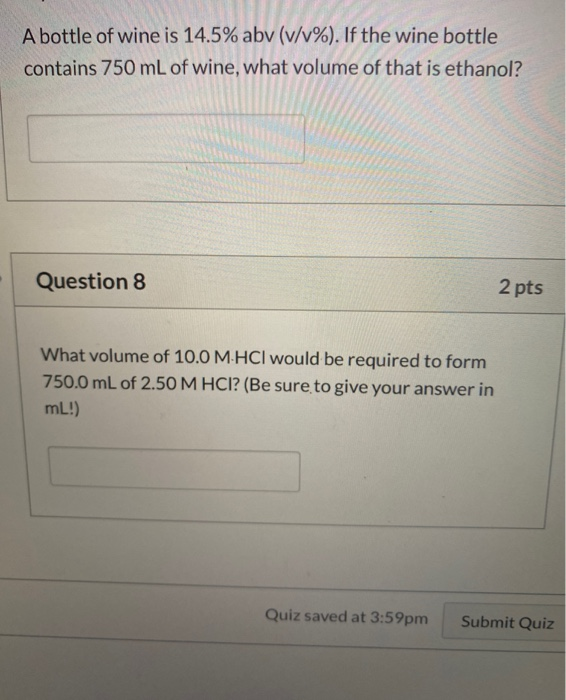A bottle of wine is 14.5% abv (v/v%). If the wine bottle contains 750 mL of wine, what volume of that is ethanol? Question 8 2 pts What volume of 10.0 M.HCl would be required to form 750.0 mL of 2.50 M HCI? (Be sure to give your answer in mL!) Quiz saved at 3:59pm Submit Quiz

• ### Principles of Inorganic Chemistry! Week 5 Effect of Temperature on Solubility of a Salt In this experiment, you w...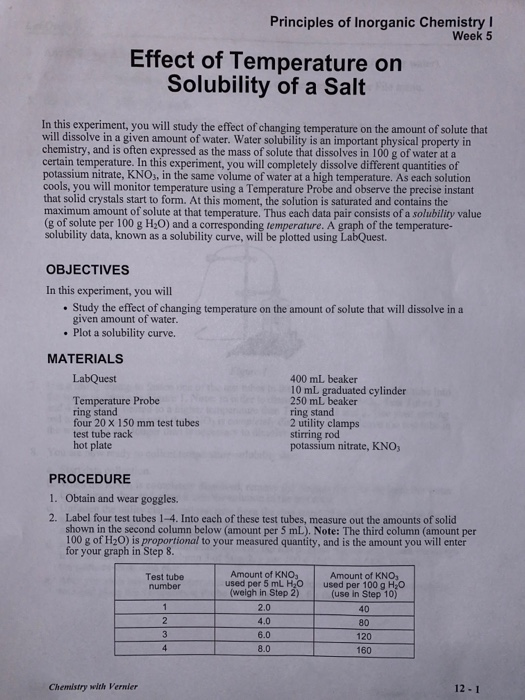Principles of Inorganic Chemistry! Week 5 Effect of Temperature on Solubility of a Salt In this experiment, you will study the effect of changing temperature on the amount of solute that will dissolve in a given amount of water. Water solubility is an important physical property in chemistry, and is often expressed as the mass of solute that dissolves in 100 g of water at a certain temperature. In this experiment, you will completely dissolve different quantities of potassium nitrate,...

• ### 1. A 19.6 gram sample of KCl is used to make a 275. mL KCl solution....

1. A 19.6 gram sample of KCl is used to make a 275. mL KCl solution. What is the molarity of the KCl solution? 2. A chemist has a bottle containing 0.25 M CuCl2. How many milliliters of this solution should the chemist use to add 12.0 grams of CuCl2 to her reaction? 3. In a saturated solution of the ionic compound sodium sulfate, Na3PO4 all of the water molecules are involved in the hydration process. some solid Na3PO4 is...

• ### CHEM-C 105 Principles of Chemistry I Summer Semester Practice Midterm Exam. 7? questions Note that questions are...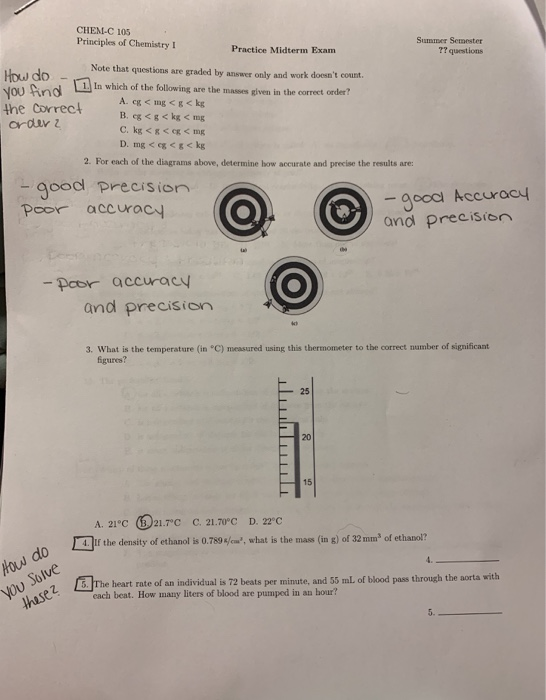CHEM-C 105 Principles of Chemistry I Summer Semester Practice Midterm Exam. 7? questions Note that questions are graded by answer only and work doesn't count HOW do You Aind 1. In which of the following are the masses given in the correct order? A. eg < mg <g< kg the Correct order 2 B. eg < g < kg < mg C. kg <g<eg < mg D. mg< eg<g< kg 2. For each of the diagrams above, determine how accurate...

• ### yes, which the data for the experiment is given in the pictures Problem #1 Use this...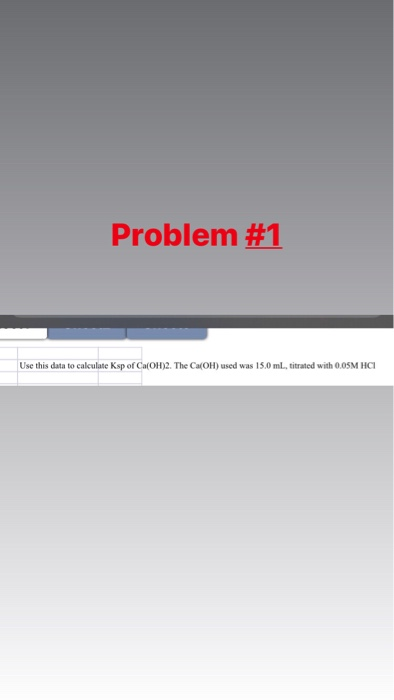yes, which the data for the experiment is given in the pictures Problem #1 Use this data to calculate Ksp of Ca(OH)2. The Ca(OH) used was 15.0 ml, titrated with 0.05M HCI DATA TABLE: TITRATION OF THE SUPERNATANT LIQUID Trial 1 Trial 2 Data Volume of CaOH, solution, mL (supernatant liquid) Initial buret reading, mL Final buret reading, mL Volume of HCl required for titration, mL Equivalence point (mL) Average Kop Problem #2 R PPPPPP LABORATORY REPORT YOU MUST SHOW...

Free Homework App

Scan Your Homework
to Get Instant Free Answers
Need Online Homework Help?

Get Answers For Free
Most questions answered within 3 hours.